As we saw from the previous tutorial on binary search trees, when insertion into a tree is not random, we can end up with a long chain of nodes stretching out to the right or left. In these cases, we lose the `n*log(n)` search performance of a BST, since we no longer split the search space in half at each branch. We could randomize insert order, but this assumes we have all the data up front, and it requires an additional step.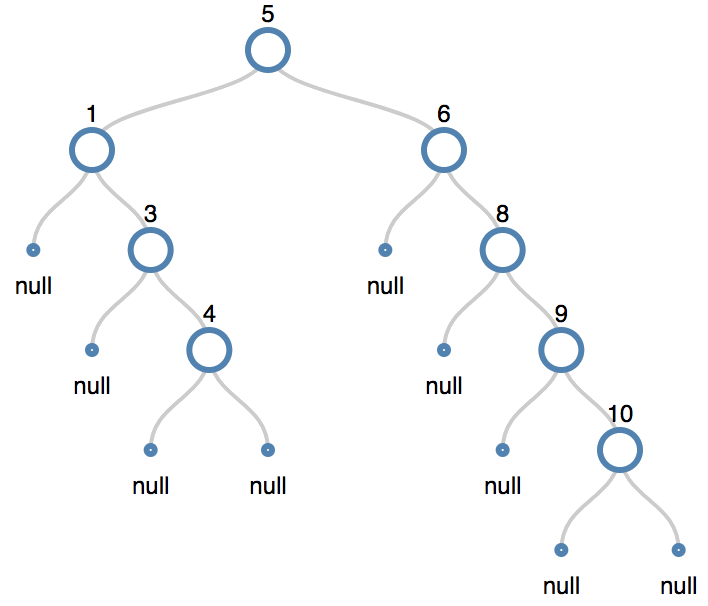##### A “degenerate” binary search tree: without balancing, sometimes the overhead to create the tree is greater than simple O(n) linear search in an array.

Wouldn’t it be nice if a tree could just balance itself regardless of insertion order?

## AVL Trees

One solution to this problem is to perform a balancing operation on the branches of the tree after we insert an item. The balancing operation is known as a rotation. Rotations reduce node height while maintaining the ordered characteristics of a binary search tree.

Remember how to search a binary search tree? At each level, we compare the search value with the node value and go right if it is greater and left if it is less. Naturally, we have one comparison per tree level. It follows that reducing height (broadening the tree out) will reduce the number of comparisons, making our search more performant.

With rotations, you can transform the tree you see above into this tree: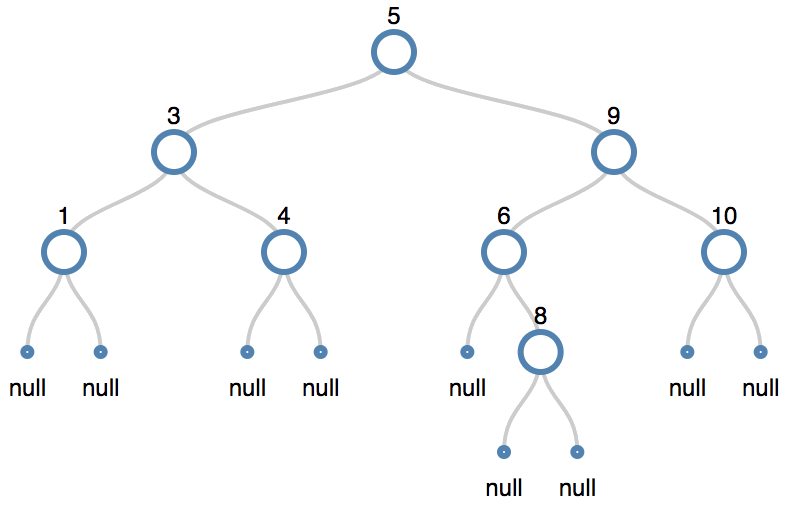It’s still in order, but now there are only 3 steps to get to 10 instead of 5. We did have to perform three operations on the tree, but assuming that we look up 10 more than once, it’ll more than pay off down the road to balance.

### Calculating Tree Height

One type balanced binary search tree is an AVL tree. It’s very expensive to keep a tree perfectly balanced at all times. The strategy here is “good enough” or satisficing: we don’t have to keep the tree perfectly balanced — let’s just not let it get out of hand. What’s out of hand? For the AVL tree, it means the difference in max height between a node’s left and right branches exceeds 1.

NB: You’ll see this a lot in algorithms: we might not care about 100% correctness (perhaps it’s even impossible), but rather that we can attain some worst-case threshold of correctness that costs less to calculate yet provides acceptable results.

We count the height of a node’s branch by calculating the maximum distance from that node to a leaf node, including the leaf node. We go down toward the leaves, counting nodes, and return the maximum distance.

We can calculate this maximum distance using a depth-first search like so:

``````function maxHeight(tree) {
if (tree==null) { return 0; }
else {
let leftD=maxHeight(tree.left);
let rightD=maxHeight(tree.right);
return leftD > rightD ? leftD+1 : rightD+1;
}
}
``````
##### We add one to the final height values to account for the null leaf nodes.

We define a Balance Factor for each node as the maximum height of the left node’s path minus the maximum height of the right node’s path.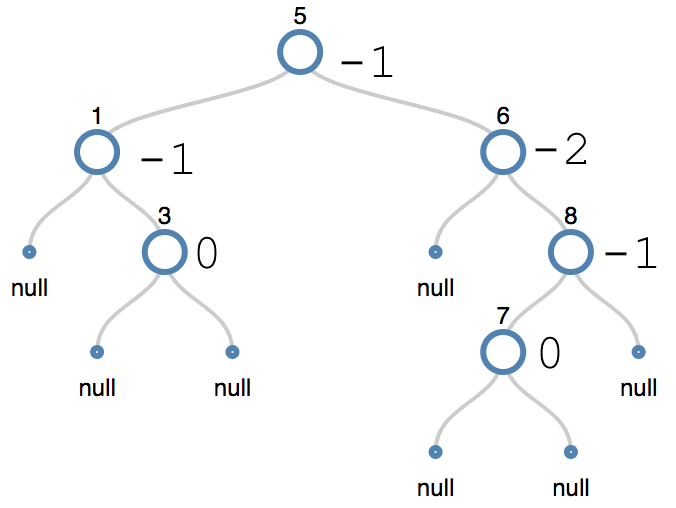##### This AVL tree is unbalanced, since the balance factor for node 6 is -2. But we can fix it!

Have a look at this AVL tree. I’ve marked the balance factor on each node. Let’s walk from the root 5 down the left branch. The longest path goes through 1, right to 3, and then down to the leaf. That’s a height of 3.

On the right branch, it goes through 6, 8, 7, and down to the leaf, a height of 4.

We calculate the balance factor for a node as Max Height[Left Branch] - Max Height[Right Branch]. In this case, it’s 3-4 or `-1`.

`0` means left and right branches are balanced. `-1` means the tree is “right heavy”, or that the right branch is longer than the left. `+1` means the tree is left-heavy: the left branch is longer than the right.

The numbers correspond to an obvious visual situation: just imagine the tree is an old-fashioned beam scale with the fulcrum at the tree’s root.

If the balance factor is outside of `[-1, 0, 1]`, we have to rebalance per AVL constraints. You see that condition on node 6. The right branch is 2x as long as the left branch, meaning that to access 7, we have to pass through extra nodes unnecessarily.

## Balancing Using Rotations

AVL trees handle these unbalanced cases through rotations. Rotations are operations that decrease the height of a given node while maintaining its ordering:

`Left Branch Value <= Node Value < Right Branch Value`

There are two directions of rotations, left and right. They can be applied in four ways:

1. Single left
2. Single right
3. Left-right (left followed by right)
4. Right-left (right followed by left)

In this tutorial we’ll cover how to code a left and right rotation and consider how to track the balance of nodes in the tree. In the next tutorial, we’ll cover how insertions and deletions can make a tree unbalanced (4 different cases), and we’ll use the code we wrote today to correct each of those four cases.

This is the generic form of a left and right rotation for a given node.##### Drawn from Introduction to Algorithms 2nd Ed by Cormen, Leiserson, Rivest, and Stein (pg. 278)

Note here that in the left rotation:

1. X keeps its left child but picks up Y’s left child on its right.
2. Y keeps its right child but takes X’s parent
3. Y becomes the node’s new root. If X didn’t have a parent node to start with (if it was the root of the entire tree), Y becomes the entire tree’s root.

Symmetrically for a right rotation:

1. Y keeps its right child but picks up X’s right child on its left.
2. X keeps its left child but takes Y’s parent
3. X becomes the node’s new root. If Y didn’t have a parent node to start with (if it was the root of the entire tree), X becomes the entire tree’s root.

It’s impossible to rotate left without a right child or rotate right without a left child. This is because right and left children in left and right rotations respectively become the new root nodes, and you cannot have null roots.

Let’s consider an example with actual values.We can fix the tree above with #4, a right-left rotation (yep, let’s just jump into the complexity first so you can see it’s not that scary).

First we rotate right about 8. That gives us this: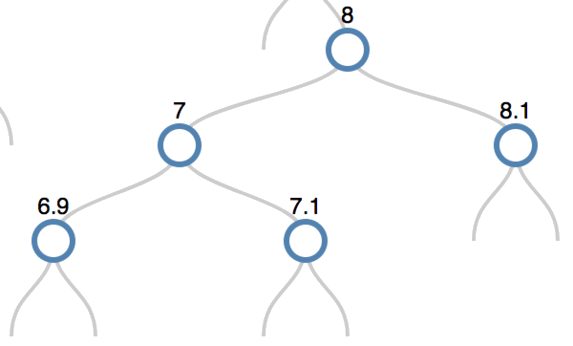=>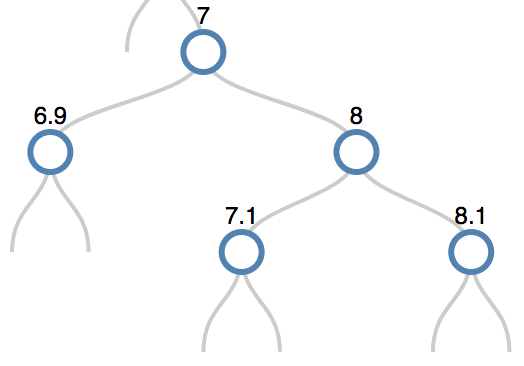##### 1. Seven keeps its left child and eight keeps its right child. 2. Seven swaps gives its right child to eight’s left child. 3. Eight gives its parent to seven, and seven picks up eight as its right child.

Note that in the diagram above, I added non-null children to the 7 and 8 so that you could see how the children swap out. I also omitted the null leaf node values for clarity.

Then we rotate left about 6. That gives us this: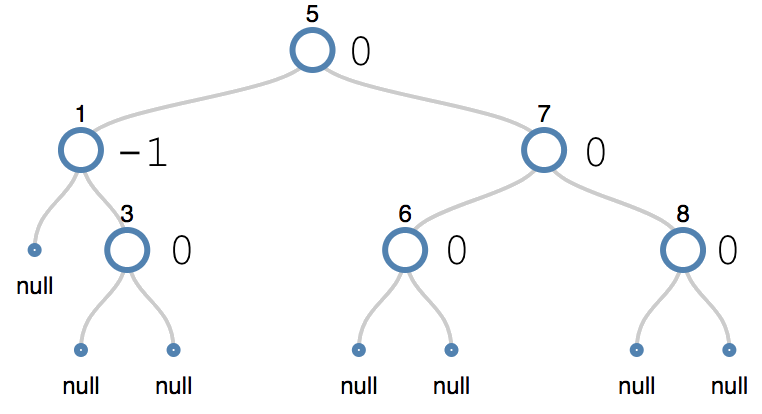Now that we’ve looked at an application of rotations, let’s code them.

## Coding Left/Right Rotations

The code for a left and right rotation is symmetric: that is, if you code one direction, just swap each direction in that function and you’ll have the opposite direction rotation. For clarity, I’m not going to reduce this behavior to a single function. Just know that you can do this and that it would be done in an optimized implementation.

### Right rotations

``````function rotateRight(tree, root) {
if (!root.left) { return false; } //the left child cannot be null
let leftChild=root.left;
root.left=leftChild.right;
let parent=findParent(tree.root, root);
if (parent===null) {
tree.root=leftChild;
} else if (parent.right===root) {
parent.right=leftChild;
} else if (parent.left===root) {
parent.left=leftChild;
}
leftChild.right=root;
}
``````

Here’s that before picture again so you can follow along with actual numbers:We’re going to rotate right about node 8.

Let’s walk through it line by line. We call the code with the tree and the root of the node we wish to rotate. If the node we are trying to rotate doesn’t have a left child, we return false. It’s not possible to perform a right rotation if the node doesn’t have a left child, because the left child becomes the new root node. You can’t have a null root.

Now we just follow the steps we saw above in the generic example.

We set `leftChild` to the left child of 8. This will be our new root node:

``````    7
/     \
6.9   7.1
``````
##### leftChild

Now we just have to reorganize this tree a little bit so that we can connect it up with 8. 8 is going to connect where 7.1 currently is, so we will swap out `root.left` with `leftChild.right`. Now we have two trees that look like this:

``````    7
/     \
6.9   7.1
``````
##### leftChild
``````    8
/     \
7.1   8.1
``````
##### root

All we have to do now is connect `leftChild` with `root`’s parent, and then connect `leftChild` to `root`.

We find the parent of the `root` (8). The parent of eight exists in the tree (you can’t see it, but it’s 6).

If the parent of eight didn’t exist and eight was the root, we set the tree root equal to `leftChild` directly.

If the parent exists, we then check to see which side of 6 eight lies on. Is it the left? Then we connect the parent’s left child to `leftChild`. Is it the right? Then we connect the parent’s right child to `leftChild`.

We have swapped out the two nodes 7 and 8. All that we have to do now is connect our original `root` to `leftChild`. If you look at this diagram, it’s obvious where we do this:

``````    7
/     \
6.9   7.1
``````
##### leftChild
``````    8
/     \
7.1   8.1
``````
##### root

We have 7.1 duplicated, so we just remove the 7.1 from `leftChild` and connect that right node to the root node with `leftChild.right=root`.

And we’re done!### Left rotations

``````function rotateLeft(tree, root) {
if (!root.right) { return false; } //we assume the right child is not null.
let rightChild=root.right;
root.right=rightChild.left;
let parent=findParent(tree.root, root);
if (parent===null) {
tree.root=rightChild;
} else if (parent.left==root) {
parent.left=rightChild;
} else if (parent.right==root) {
parent.right=rightChild;
}
rightChild.left=root;
}
``````

We’ll walk through the code here for a left rotation with the diagram as well (even though it’s just a mirror image of the right rotation).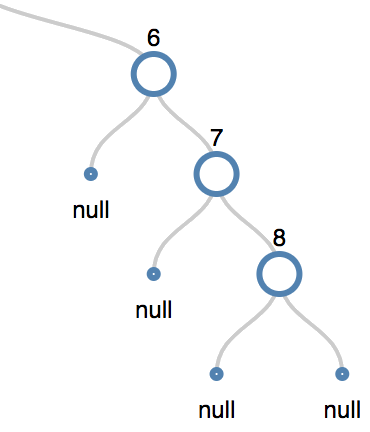We’re going to rotate left about node 6.

Let’s walk through it line by line. We call the code with the tree and the root of the node we wish to rotate. If the node we are trying to rotate doesn’t have a right child, we return false. It’s not possible to perform a left rotation if the node doesn’t have a right child, because the right child becomes the new root node. You can’t have a null root.

Now we just follow the steps we saw above in the generic example.

We set `rightChild` to the right child of the root. This will be our new root node:

``````     7
/      \
null     8
``````
##### rightChild

Now we just have to reorganize this tree a little bit so that we can connect it up with 6. 6 is going to connect where the null currently is, so we will swap out `root.right` with `rightChild.left`. Now we have two trees that look like this:

``````    7
/     \
null    8
``````
##### rightChild
``````    6
/     \
null   null
``````
##### root

All we have to do now is connect `rightChild` with `root`’s parent, and then connect `rightChild` to `root`.

We find the parent of the `root` (6). The parent of six exists in the tree (you can’t see it, but it’s 5).

If the parent of six didn’t exist and seven was going to be the new root, we set the tree root equal to `rightChild` directly.

If the parent exists, we then check to see which side of 5 six lies on. Is it the left? Then we connect the parent’s left child to `rightChild`. Is it the right? Then we connect the parent’s right child to `rightChild`.

We have swapped out the two nodes 6 and 7. All that we have to do now is connect our original `root` to `rightChild`. If you look at this diagram, it’s obvious where we do this:

``````    7
/     \
null    8
``````
##### rightChild
``````    6
/     \
null   null
``````
##### root

We have the open spot on `rightChild`’s left, so we just replace its null with `root` by connecting that left node to the root node with `rightChild.left=root`.

And we’re done!

## Confused Yet?

If you find all this talk about node rotation confusing, please don’t worry. It’s not so intuitive. Try drawing it out and then coding it as you draw it. I still have to draw it out. Otherwise I’ll end up coding circular references that recurse infinitely and overflow the call stack when I try to depth-first traverse. Don’t worry, we’ve all been there.

Just remember the basics of rotation. Rotation helps us achieve the following objectives:

• preserve the ordering of a binary search tree
• reduce branches’ height to reduce the number of comparisons needed to search for a value

## Next steps

Now that we have the concepts of node balance factors, the AVL method of minimizing the node height differential to one of `[-1, 0, 1]`, and rotation, we can incorporate these tools to rebalance a given node when we perform insertions or deletions on our tree.

Since this tutorial was already quite a lot to digest, that’ll wait until part two. We’ve coded all the tools we need to perform the rebalancing. We only have to incorporate them into tree methods that we’ve already defined. Try to think about how it’ll work!